• 主要为大家详细介绍了Opencv绘制最小外接矩形最小外接圆的方法，具有一定的参考价值，感兴趣的小伙伴们可以参考一下
• 资源中包含了两个m文件。...minboundrect.m用于绘制运动目标的最小外接矩形框，可任意设置最小外接矩形框的角度。 本人做的是视频中运动车辆的检测，读者可自行更换检测算法以及参数调整以匹配不同的运动场景。
• 本代码是本人亲自写的，描写了一个如何把点放在一个矩形的面积下使得矩形面积最小代码
• //将最小矩形的四个顶点以Point的形式放置在vector容器中 } ///=============【2】计算最小轮廓的面积和长度============ double recArea = contourArea ( min_rectangle ) ; double ...


版权声明：本文为博主原创文章，遵循 CC 4.0 BY-SA 版权协议，转载请附上原文出处链接和本声明。

本文链接：
https://blog.csdn.net/sinat_34707539/article/details/52057844

本文将介绍OpenCV计算物体最小包围矩形面积和周长
两个函数：contourArea()和arcLength
知识点：
1.寻找最小包围矩形 2.计算最小轮廓的面积和长度 3.定义和输出vector容器点坐标
代码示例：
//寻找最小包围矩形
//计算最小轮廓的面积和长度
//定义和输出vector容器点坐标

#include <opencv2/opencv.hpp>
#include "opencv2/highgui/highgui.hpp"
#include "opencv2/imgproc/imgproc.hpp"
#include <vector>
using namespace std;
using namespace cv;

int main(int argc, char** argv)
{
Mat testImage(600, 600, CV_8UC3);//画布大小600x600
RNG& rng = theRNG();
//按回车键一直更新
while (1)
{
cout << "===========Begin============\n" << endl;
testImage = Scalar::all(0);//将画布设置为黑色，每次按键后可更新
//int count = (unsigned)rng % 100 + 8;//随机生成点的数量
int count = rng.uniform(4, 6);//随机产生点的个数
cout << "共产生 " << count << " 个随机点" << endl;
cout << "各点坐标如下：" << endl;//输出产生随机点个数

vector<Point>points;//vector容器存放点坐标

for (int i = 0; i < count; i++)
{
Point point;
//点坐标随机产生
point.x = rng.uniform(testImage.cols / 5, testImage.cols * 4 / 5); //横坐标x在范围（600/1，600*4/5）随机产生
point.y = rng.uniform(testImage.rows / 4, testImage.rows * 3 / 4);//纵坐标y在范围（600/4，600*3/4）随机产生
points.push_back(point);
cout << "Point" << i + 1 << ": " << point << endl;//依次输出随机产生每个点的坐标
}

//输出并标记各点坐标
cout << "基于Mat的vector:\n" << Mat(points) << endl;//基于Mat类的vector将以矩阵形式输出坐标
for (int i = 0; i < count; i++)
circle(testImage, points[i], 5, Scalar(255, 0, 255), -1, 4);//以随机点为圆心，画出半径为5的实心圆

//=============【1】寻找最小包围矩形================
RotatedRect minRect = minAreaRect(Mat(points));
Point2f vertex;//用于存放最小矩形的四个顶点
minRect.points(vertex);//返回矩形的四个顶点给vertex
//绘制最小面积包围矩形
vector<Point>min_rectangle;
for (int i = 0; i < 4; i++)
{
line(testImage, vertex[i], vertex[(i + 1) % 4], Scalar(0, 255, 0), 1, 8);//非常巧妙的表达式
min_rectangle.push_back(vertex[i]);//将最小矩形的四个顶点以Point的形式放置在vector容器中
}

///=============【2】计算最小轮廓的面积和长度============
double recArea=contourArea(min_rectangle);
double recPerimeter = arcLength(min_rectangle,true);

//输出
cout << "最小包围矩形的面积: " << recArea << endl;
cout << "最小包围矩形的周长: " << recPerimeter<< endl;
cout << "\n===========End============\n" << endl;
imshow("最小包围矩形的面积和长度", testImage);
char key;
key = (char)waitKey();
if (key == 27 || key == 'q' || key == 'Q')//按下ESC 或q 或 Q 退出
break;

}
return 0;
}

运行结果：
情形一：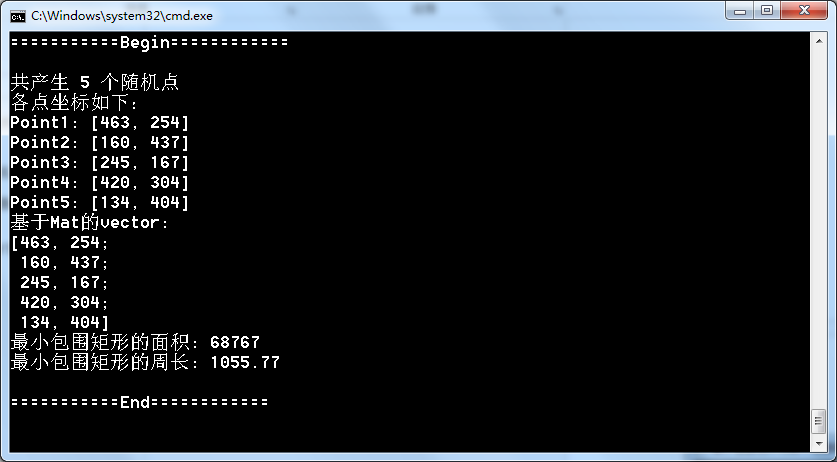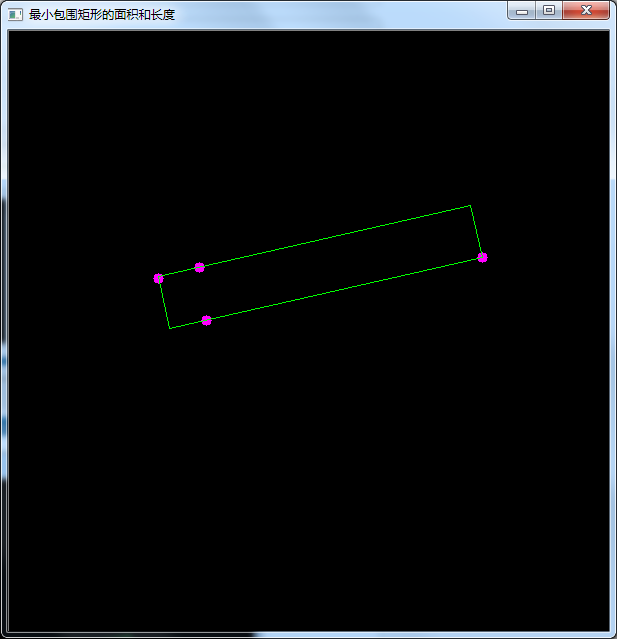情形二：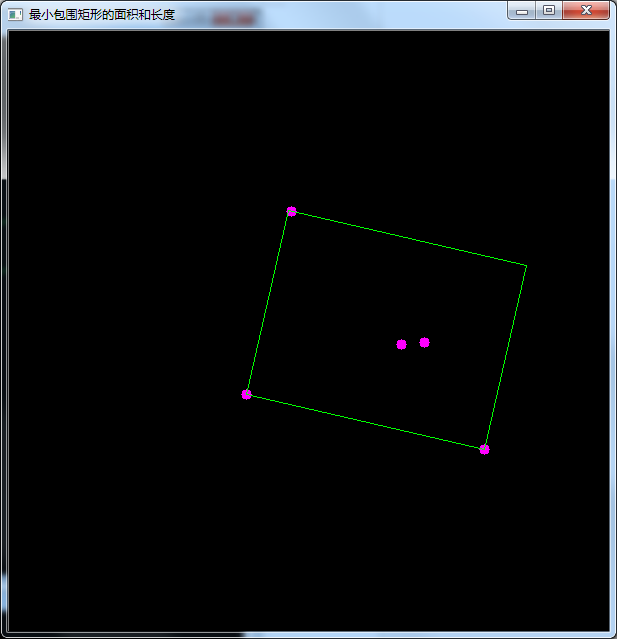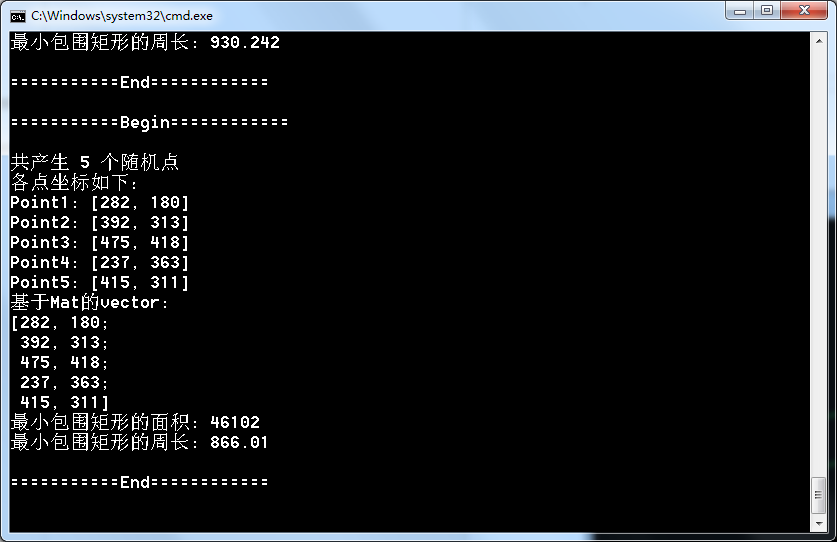展开全文opencv
• 1.VS2015+Halcon联合编程, 2.窗口句柄Halcon, 3.C#中读取图片并框选区域， 4.将官方发布的代码精简过，分成两个类，方便调用和演示 5.Halcon库文件是12版本的
• 求多个目标的最小外接矩形，有说明，matlab版
• 在openCV中，使用最小外接矩形来定位目标是一个很常用的功能。有时，我们需要把矩形的中心和方向展示出来，这时，可以使用cv2.arrowedLine（）来实现这一目标。具体实现方法如下： 首先，我们需要使用cv2....
        在openCV中，使用最小外接矩形来定位目标是一个很常用的功能。有时，我们需要把矩形的中心和方向展示出来，这时，可以使用cv2.arrowedLine（）来实现这一目标。具体实现方法如下：
首先，我们需要使用cv2.minAreaRect来获取最小外接矩形的参数，例如
rect = cv2.minAreaRect(contours[max_index])
获取到的rect结果为（（中心点坐标），（宽度，高度），角度）。得到的结果中的宽度和高度并不是按照长短来划分的，而是旋转矩形中接近水平的边为宽度值，接近垂直的边为长度值。rect中角度指的是接近水平的边与水平线的夹角。而通常我们所需要的是“较长边为长度，较短边为宽度，沿长边方向是外接矩形的方向”。所以，接下来我们需要对这个情况做出修改。当发生长度值小于宽度值的情况时，我们就把长宽值交换，并且把角度增加90度。这时，所有的oritation都是与短边平行的，我们再次把角度增加90度，即可实现所有oritation都与长边平行。最后，把角度统一保留至0-180.
    if(width > length):
width,length = length,width
oritation = oritation + 90
oritation = oritation + 90
if(oritation > 180):
oritation = oritation - 180
获取了外接矩形的oritation后，我们就可以将区域center作为箭头的起点，按照oritation方向，计算长度为L的箭头的终点坐标。
                    arrow_end_x = int(x + math.cos(oritation / 180 * 3.14) * L)
arrow_end_y = int(y + math.sin(oritation / 180 * 3.14) * L)
最后，使用cv2. arrowedLine()来画出箭头。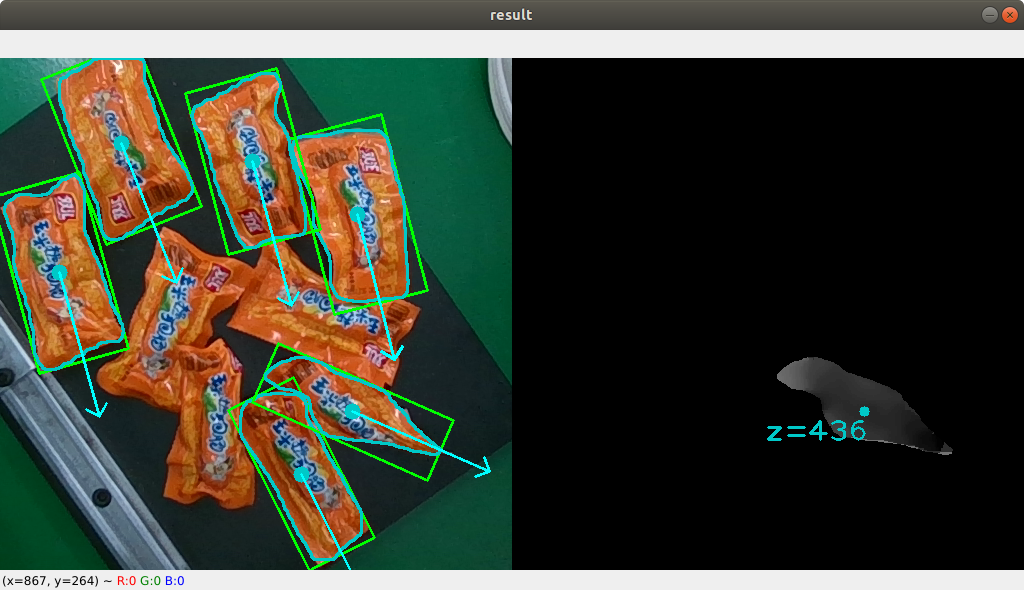展开全文• 在图像裁剪操作中，opencv和pillow两个库都具有相应的函数，但是这两个库中的函数仅仅能对与图片平行的矩形进行裁剪操作，如果想要对目标的最小外接矩形进行裁剪该如何操作呢？如下所示： 具体处理该问题的思路如下...python 图片
• 对于一个倾斜的矩形或不规则单连通域，找出其最小矩形，并将其旋转到X,Y坐标系平行位置。如：将 下图蓝色矩形区范围内的黑色区域旋转至，蓝色矩形的边平行于X，Y坐标系。 代码1 图像以固定的步幅进行旋转，每旋转一...
对于一个倾斜的矩形或不规则单连通域，找出其最小矩形，并将其旋转到X,Y坐标系平行位置。如：将 下图蓝色矩形区范围内的黑色区域旋转至，蓝色矩形的边平行于X，Y坐标系。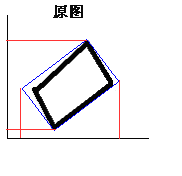代码1
图像以固定的步幅进行旋转，每旋转一次，获取X,Y轴上的投影长度，相乘得出投影矩形面积。找出旋转过程中最小的投影面积，此时就是最小矩形。 S=（X2-X1）*(Y2-Y1) 方案1：直接对图像进行旋转，并不断的计算投影面积。
%% 代码作用：找出图像的最小矩形，并将图像旋转到最小矩形在x,y轴方向
% 输入图像仅包含一个单连通域的图形，否则可能出错；
% 思路：
%       1.将图像在90°内旋转，找出在X,Y坐标上投影面积最小时的角度即可；
%       2.先找出最小矩形，然后判断最小矩形和X,Y轴的夹角，然后旋转一下即可。
% 下面代码采用第一种方法进行。
% 步骤：
%       1.二值化图像；2.边旋转边检查投影面积；
% 作者：cw      时间：2019.8.12
clc;
clear all;
t0 = clock;
%% 图像读入，灰度化，二值化，显示二值图
img = rgb2gray(img);
BW=~im2bw(img);
figure;imshow(BW);

%% 计算间隔1°旋转过程中，图像的X,Y投影面积
Area = AreaCheck_PlanB(BW);
Area_min = min(Area);
% 找出最小面积时旋转的角度,注意Ind中可能包含好几个索引，
% 即在旋转过程中可能有好几个角度都能够使得投影面积最小
Ind = find(Area == Area_min);
Ind_first = Ind(1,1);

%% 将图形旋转到X,Y局部坐标系，绘制旋转后的图像
Rota_F = imrotate(BW,Ind_first,'nearest');
figure;
imshow(Rota_F);
%% 存在问题
% 经过转动后的图形中直线不是直线了，有点误差o(╥﹏╥)o
% 原因：1 旋转角度可能有微小差异，需要抖动处理；
Time_exhuasted = etime(clock,t0);

AreaCheck_PlanB.m文件：
function area = AreaCheck_PlanB(Image_BW)
% 作用：将图像轮廓，每间隔1°进行一次旋转，并计算每次旋转后轮廓在X,Y坐标轴上的投影面积
% Image_Outline：轮廓坐标，是一个m*2的矩阵，第一列存放x坐标，第二列存放y坐标
%          area：是一个90*1的向量，里面存储每个旋转角度下的投影面积
% imrotate()中采用的的角度制；
area = zeros(90,1);
X_MAX=0;
X_MIN=0;
Y_MAX=0;
Y_MIN=0;

for i=1:90
Rotate_new = imrotate(Image_BW,i,'nearest');
% 计算X,Y投影面积并保存到area
H = size(Rotate_new,1);
% 找出X_MIN和X_MAX
for j=1:H
% 从上到下，逐行寻找等于1的行
if max(Rotate_new(j,:))==1
X_MIN = j;
break;
end
end
for j=1:H
% 从下到上，逐行寻找等于1的行
if max(Rotate_new(H+1-j,:))==1
X_MAX = H+1-j;
break;
end
end

L = size(Rotate_new,2);
% 找出Y_MIN和Y_MAX
for k=1:L
% 从左到右，逐行寻找等于1的列
if max(Rotate_new(:,k))==1
Y_MIN = k;
break;
end
end
for k=1:L
% 从右到左，逐行寻找等于1的列
if max(Rotate_new(:,L+1-k))==1
Y_MAX = L+1-k;
break;
end
end
area(i,1)=(X_MAX-X_MIN)*(Y_MAX-Y_MIN);
end
end

代码2
方案2：代码是先提取出轮廓，旋转轮廓并计算面积，找出旋转角度，最后按照找出的角度对图像进行旋转。
%% 代码作用：找出图像的最小矩形，并将图像旋转到最小矩形在x,y轴方向
% 输入图像仅包含一个单连通域的图形，否则可能出错；
% 思路：
%       1.将图像在正负45°内旋转，找出在X,Y坐标上投影面积最小时的角度即可；
%       2.先找出最小矩形，然后判断最小矩形和X,Y轴的夹角，然后旋转一下即可。
% 下面代码采用第一种方法进行。
% 步骤：
%       1.二值化图像；2.边旋转边检查投影面积；
% 作者：cw      时间：2019.8.12
clc;
clear all;

%% 图像读入，灰度化，二值化，显示二值图
img = rgb2gray(img);
BW=~im2bw(img);
figure;imshow(BW);

%% 连通域检测，B的每个元素表示一个轮廓，L是每个像素所属区域的标记
[B,L]=bwboundaries(BW);
% 将所有边缘点都存入一个矩阵,B的每个元素都是X*2矩阵，即每一行都存的x,y的坐标值
% 当B有多个元素时，cell2mat()函数将B中的每个元素矩阵按照行方向连接
% 例如：B(1)是m*2,B(2)是n*2,那么变换后的LB就是(m+n)*2
LB=cell2mat(B);

%% 计算间隔1°旋转过程中，图像的X,Y投影面积
Area = AreaCheck(LB);
Area_min = min(Area);
% 找出最小面积时旋转的角度,注意Ind中可能包含好几个索引，
% 即在旋转过程中可能有好几个角度都能够使得投影面积最小
Ind = find(Area == Area_min);
%% 将图形旋转到X,Y局部坐标系
% 坐标变换矩阵
Ind_first = Ind(1,1);
Rota_F =[cos(Ind_first),sin(Ind_first); -sin(Ind_first),cos(Ind_first)];
% 轮廓坐标变换
Outline_F = round(LB*Rota_F);

%% 绘制旋转后的图像
% 注意：imrotate()函数中使用的是角度制，AreaCheck()函数使用的是弧度制
% Ind_first正好对应的角度
Image_F = imrotate(BW,Ind_first,'nearest');
figure;
imshow(Image_F);
%% 存在问题
% 经过转动后的图形中直线不是直线了，有点误差o(╥﹏╥)o
% 原因：1 旋转角度可能有微小差异，需要抖动处理；2 计算过程中精度损失；

AreaCheck.m 中包含的一个函数，该函数执行图像轮廓的旋转变换和求投影面积。
function area = AreaCheck( Image_Outline )
% 作用：将图像轮廓，每间隔1°进行一次旋转，并计算每次旋转后轮廓在X,Y坐标轴上的投影面积
% Image_Outline：轮廓坐标，是一个m*2的矩阵，第一列存放x坐标，第二列存放y坐标
%          area：是一个90*1的向量，里面存储每个旋转角度下的投影面积
% 采用rotate（）显然不适宜，因为这是对整个图像进行旋转，这里我们只是需要对轮廓坐标进行旋转
% 所以这里手动进行坐标变换,注意这里是顺时针旋转
area = zeros(90,1);
for i=1:90
% 坐标变换矩阵,这里是弧度制不是角度制
angle = i*pi/180;
Rota=[cos(angle),sin(angle); -sin(angle),cos(angle)];
% 轮廓坐标变换,坐标值取四舍五入的整数
Outline_new = round(Image_Outline*Rota);
% 计算X,Y投影面积并保存到area
X_MAX=max(Outline_new(:,1));
X_MIN=min(Outline_new(:,1));
Y_MAX=max(Outline_new(:,2));
Y_MIN=min(Outline_new(:,2));
area(i,1)=(X_MAX-X_MIN)*(Y_MAX-Y_MIN);
end
end

这里为了描述基本原理，故而代码比较简单明了，但是效率低下，可根据需要利用优化算法进行快速计算。
效果：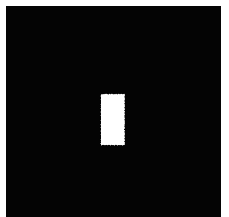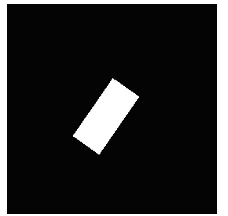上图左右位置 反了，阅读时需要注意。 两个方案的代码运行速度有明显的差距，方案1运行时间6.6秒，方案2运行时间1.7秒，总体而已速度很慢。方案2代码尚有很大的优化空间，闲暇时再进行代码优化。
展开全文MATLAB
• 绘制轮廓的最小外接斜矩形最小外接正矩形最小外接圆，并将结果输出到原图像上，可参考博客：https://blog.csdn.net/didi_ya/article/details/113863388
• C++ OpenCV4绘制轮廓最小外接矩形使用方法发生了一些变化： OpenCV2/3的代码： findContours(srcImg, contours, hierarcy, CV_RETR_EXTERNAL, CV_CHAIN_APPROX_NONE); cout<<"num="<<contours.size...
C++ OpenCV4绘制轮廓最小外接矩形使用方法发生了一些变化：
OpenCV2/3的代码：
findContours(srcImg, contours, hierarcy, CV_RETR_EXTERNAL, CV_CHAIN_APPROX_NONE);
cout<<"num="<<contours.size()<<endl;
vector<Rect> boundRect(contours.size());  //定义外接矩形集合
vector<RotatedRect> box(contours.size()); //定义最小外接矩形集合
Point2f rect;
for(int i=0; i<contours.size(); i++)
{
box[i] = minAreaRect(Mat(contours[i]));  //计算每个轮廓最小外接矩形
boundRect[i] = boundingRect(Mat(contours[i]));

circle(dstImg, Point(box[i].center.x, box[i].center.y), 5, Scalar(0, 255, 0), -1, 8);
//绘制最小外接矩形的中心点
box[i].points(rect);  //把最小外接矩形四个端点复制给rect数组

rectangle(dstImg, Point(boundRect[i].x, boundRect[i].y), Point(boundRect[i].x +
boundRect[i].width, boundRect[i].y + boundRect[i].height), Scalar(0, 255, 0), 2, 8);
for(int j=0; j<4; j++)
{
line(dstImg, rect[j], rect[(j+1)%4], Scalar(0, 0, 255), 2, 8);  //绘制最小外接矩形每条边
}

}	
OpenCV4的代码(区别部分)
RotatedRect box = minAreaRect(Mat(contours));  //计算每个轮廓最小外接矩形
vector<Point2f> boxPts(4);
box.points(boxPts.data());

for (int j = 0; j < 4; j++)
{
line(ROI, boxPts[j], boxPts[(j + 1) % 4], Scalar(0, 0, 255), 2, 8);  //绘制最小外接矩形每条边
}

展开全文• RotatedRect box=minAreaRect(Mat(contours[i]));//第i个轮廓 Point2f vertex; box.points(vertex); for(int i=0;i ...line(w_map,vertex[i],vertex[(i+1)%4],Scalar(100,200,211),1,CV_AA);...}opencv
• 主要介绍了Python实现图片查找轮廓、多边形拟合、最小外接矩形代码，具有很好的参考价值，希望对大家有所帮助。一起跟随小编过来看看吧
• 方法主要是利用面积最小实现。代码如下：function main() I=imread('4.jpg'); bw=im2bw(I); [r c]=find(bw==1);... % 'a'是按面积算的最小矩形，如果按边长用'p' imshow(bw);hold on line(rectx...
• 绘制如上图片的最小内接矩阵： def draw_min_rect_rectangle(mask_path): image = cv2.imread(mask_path) thresh = cv2.Canny(image, 128, 256) contours, hierarchy = cv2.findContours(thresh, cv2.RETR_...opencv 计算机视觉 canvas
• cv2.boundingRect(img)函数 ...然后利用cv2.rectangle(img, (x,y), (x+w,y+h), (0,255,0), 2),画出矩形 参数解释 第一个参数：img是原图 第二个参数：（x，y）是矩阵的左上点坐标 第三个参数：（x...OpenCV 图像处理 cv2 边缘检测
• matlab实现的，画最小外接矩形。其中包括求出最小外接矩形的四个顶点坐标，周长，面积等参数。
• Opencv绘制最小外接矩形最小外接圆   Opencv中求点集的最小外结矩使用方法minAreaRect，求点集的最小外接圆使用方法minEnclosingCircle。 minAreaRect方法原型： RotatedRect minAreaRect( InputArray ...
• min_rect = cv2.minAreaRect(coords)//由点集获取最小矩形（包含中心坐标点、宽和高、偏转角度） print("min_rec =",min_rect) box = cv2.boxPoints(min_rect)//获取最小矩形的4个顶点坐标。 但是通过一下这个绘制...
• 所用函数： ...cv2.minAreaRect —— 找到最小外接矩形矩形具有一定的角度） cv2.boxPoints —— 外接矩形的坐标位置 cv2.drawContours(image, [box], 0, (0, 0, 255), 3) —— 根据点画出矩形 # -*- cod
• 该篇博客是记录并分享使用C++ opencv绘制旋转矩形，该代码可以实时显示旋转的过程。 首先贴出效果图： 蓝色—原始矩形 绿色—旋转矩形 白色—旋转矩形的外接矩形 下面是代码： // opencv_study.cpp : 定义控制台...opencv c++
• #include "iostream"; #include "opencv.hpp" ...//绘制最小外接矩形的每条边 } } namedWindow("结果图", 1); imshow("结果图", resultImage); waitKey(0); return 0; } 运行结果：opencv c++
• 对下面这张图像，检测其边界框、最小矩形区域以及最小闭圆的轮廓。 hammer.jpg 使用cv2.pyrDown()函数缩小图片。 使用cv2.threshold()函数进行二值化处理。 使用cv2.findContours()函数检测轮廓。 使用cv...OpenCV 轮廓检测 图像处理 Python
• #边界框，最小矩形区域和最小闭圆的轮廓 '''cv2.pyrUp()和cv2.pyrDown()用于构建图像金字塔 一般情况下，我们要处理是一副具有固定分辨率的图像,但是有些情况下， 需要对同一图像的不同分辨率的子图像进行处理。 ...OpenCV 边缘检测 轮廓 深度学习
• 这只是针对某一个轮廓获取最小外接矩形，如果想要获取图像所有轮廓的最小外接矩形则需要将所有轮廓的点集合合并到一个np.array中： allarea = np.concatenate(contours)#合并所有轮廓点集合 rect = cv2....数据预处理 python 轮廓检测
•OpenCV...